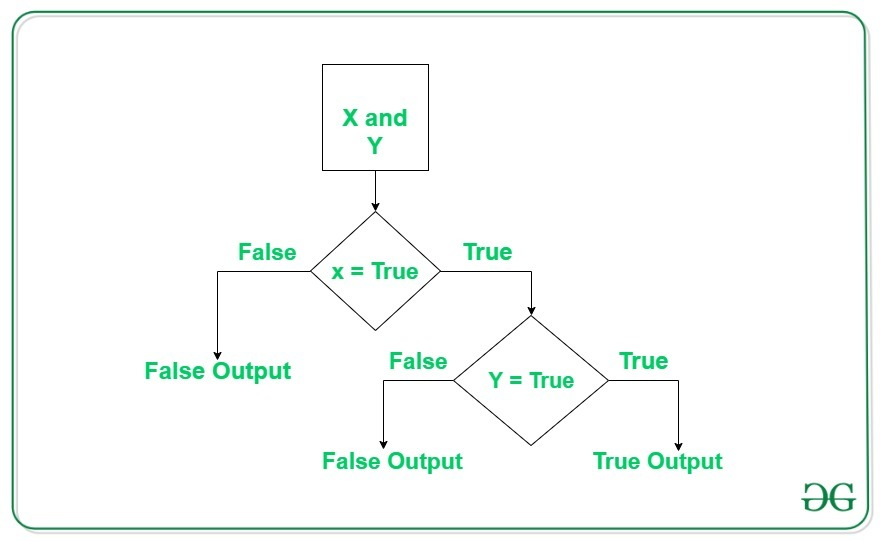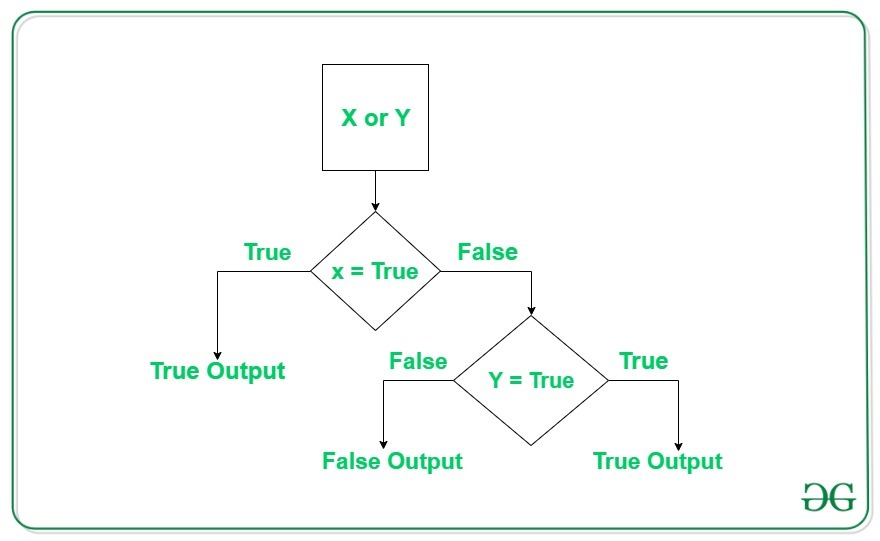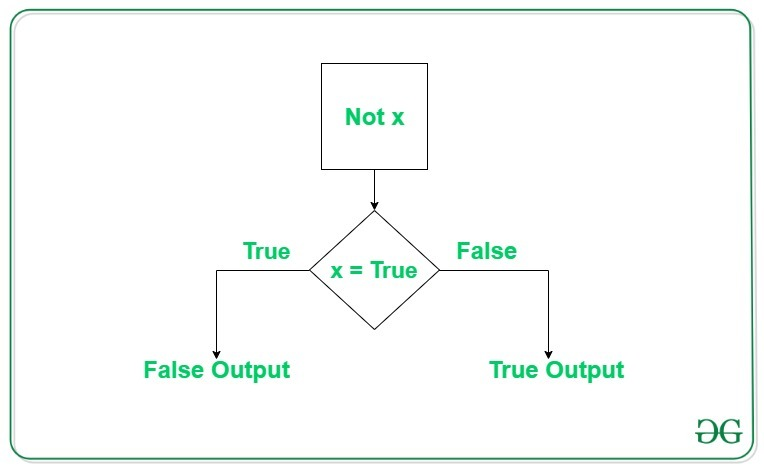# Python Logical Operators with Examples

## Python Logical Operators with Examples

Operators are used to perform operations on values and variables. These are the special symbols that carry out arithmetic and logical computations. The value the operator operates on is known as Operand.

## Logical operators

In Python, Logical operators are used on conditional statements (either True or False). They perform Logical ANDLogical OR and Logical NOT operations.

OPERATOR DESCRIPTION SYNTAX
and Logical AND: True if both the operands are true x and y
or Logical OR: True if either of the operands is true x or y
not Logical NOT: True if operand is false not x

#### Logical AND operator

Logical operator returns `True `if both the operands are True else it returns `False`.Example #1:

 `# Python program to demonstrate` `# logical and operator` ` ` `a ``=` `10` `b ``=` `10` `c ``=` `-``10` ` ` `if` `a > ``0` `and` `b > ``0``:` `    ``print``(``"The numbers are greater than 0"``)` ` ` `if` `a > ``0` `and` `b > ``0` `and` `c > ``0``:` `    ``print``(``"The numbers are greater than 0"``)` `else``:` `    ``print``(``"Atleast one number is not greater than 0"``)`

Output:

```The numbers are greater than 0
Atleast one number is not greater than 0
```

Example #2:

 `# Python program to demonstrate` `# logical and operator` ` ` `a ``=` `10` `b ``=` `12` `c ``=` `0` ` ` `if` `a ``and` `b ``and` `c:` `    ``print``(``"All the numbers have boolean value as True"``)` `else``:` `    ``print``(``"Atleast one number has boolean value as False"``)`

Output:

```Atleast one number has boolean value as False
```

Note: If the first expression evaluated to be false while using and operator, then the further expressions are not evaluated.

#### Logical or operator

Logical or operator returns True if either of the operands is True.Example #1:

 `# Python program to demonstrate` `# logical or operator` ` ` `a ``=` `10` `b ``=` `-``10` `c ``=` `0` ` ` `if` `a > ``0` `or` `b > ``0``:` `    ``print``(``"Either of the number is greater than 0"``)` `else``:` `    ``print``(``"No number is greater than 0"``)` ` ` `if` `b > ``0` `or` `c > ``0``:` `    ``print``(``"Either of the number is greater than 0"``)` `else``:` `    ``print``(``"No number is greater than 0"``)`

Output:

```Either of the number is greater than 0
No number is greater than 0
```

Example #2:

 `# Python program to demonstrate` `# logical and operator` ` ` `a ``=` `10` `b ``=` `12` `c ``=` `0` ` ` `if` `a ``or` `b ``or` `c:` `    ``print``(``"Atleast one number has boolean value as True"``)` `else``:` `    ``print``(``"All the numbers have boolean value as False"``)`

Output:

```Atleast one number has boolean value as True
```

Note: If the first expression evaluated to be True while using or operator, then the further expressions are not evaluated.

#### Logical not operator

Logical not operator work with the single boolean value. If the boolean value is `True `it returns `False `and vice-versa.Example:

 `# Python program to demonstrate` `# logical not operator` ` ` `a ``=` `10` ` ` `if` `not` `a:` `    ``print``(``"Boolean value of a is True"``)` ` ` `if` `not` `(a``%``3` `=``=` `0` `or` `a``%``5` `=``=` `0``):` `    ``print``(``"10 is not divisible by either 3 or 5"``)` `else``:` `    ``print``(``"10 is divisible by either 3 or 5"``)`

Output:

```10 is divisible by either 3 or 5
```

## Order of evaluation of logical operators

In the case of multiple operators, Python always evaluates the expression from left to right. This can be verified by the below example.

Example:

 `# Python program to demonstrate` `# order of evaluation of logical ` `# operators` ` ` `def` `order(x):` `    ``print``(``"Method called for value:"``, x)` `    ``return` `True` `if` `x > ``0` `else` `False` `     ` `a ``=` `order` `b ``=` `order` `c ``=` `order` ` ` `if` `a(``-``1``) ``or` `b(``5``) ``or` `c(``10``):` `    ``print``(``"Atleast one of the number is positive"``)`

Output:

```Method called for value: -1
Method called for value: 5
Atleast one of the number is positive
```

Last Updated on October 28, 2021 by admin

## How to Run a Python ScriptHow to Run a Python Script

How to Run a Python Script Python is a well known high-level programming language. The Python

## Top 10 Python IDE and Code Editors in 2020Top 10 Python IDE and Code Editors in 2020

Top 10 Python IDE and Code Editors in 2020 Python… the most popular and favorite programming

## How to get column and row names in DataFrame?How to get column and row names in DataFrame?

Get column and row names in DataFrame   While analyzing the real datasets which are

## Python – os.remove() methodPython – os.remove() method

Python | os.remove() method OS module in Python provides functions for interacting with the operating system.

## Create a Pandas DataFrame from List of DictsCreate a Pandas DataFrame from List of Dicts

Pandas DataFrame is a 2-dimensional labeled data structure with columns of potentially different types. It

## Linear Regression – Python ImplementationLinear Regression – Python Implementation

Linear Regression (Python Implementation) This article discusses the basics of linear regression and its implementation

## Extract numbers from a text file and add them using PythonExtract numbers from a text file and add them using Python

Extract numbers from a text file and add them using Python Python too supports file

## What is the maximum possible value of an integer in Python ?What is the maximum possible value of an integer in Python ?

What is the maximum possible value of an integer in Python ? Consider below Python